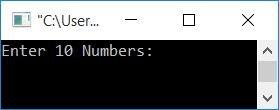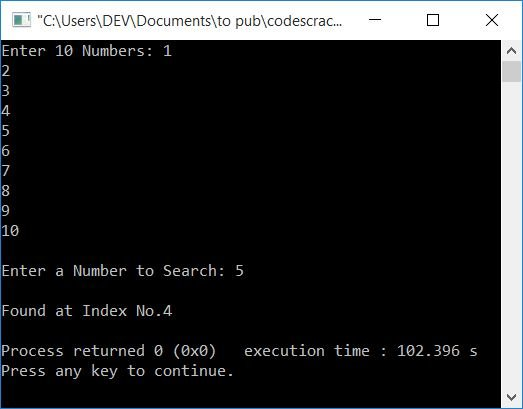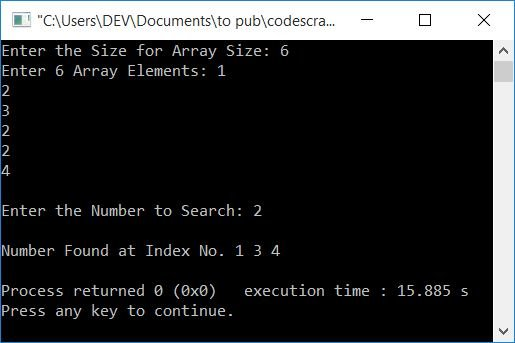# C++ Program for Linear Search

In this article, you will learn and get code to implement linear search in C++. Here is the list of programs on linear search available in this article:

If you're not familiar with the logic used behind linear search, you can refer to the linear search algorithm and examples to get all the information you need before going through these programs.

## Linear Search in C++

To search any element present inside the array in C++ programming using the linear search technique, you have to ask the user to enter any 10 numbers as 10 array elements, and then ask them to enter a number to search, as shown in the program given below.

This program doesn't allow the user to define the size of an array. Later on, you will go through the program that allows the user to define the size and also prints all the indexes of an element if it is found multiple times.

This is the simplest program to implement linear search in C++.

```#include<iostream>
using namespace std;
int main()
{
int arr, i, num, index;
cout<<"Enter 10 Numbers: ";
for(i=0; i<10; i++)
cin>>arr[i];
cout<<"\nEnter a Number to Search: ";
cin>>num;
for(i=0; i<10; i++)
{
if(arr[i]==num)
{
index = i;
break;
}
}
cout<<"\nFound at Index No."<<index;
cout<<endl;
return 0;
}```

This program was built and runs under the Code::Blocks IDE. Here is its sample run:Now supply any 10 numbers, say 1, 2, 3, 4, 5, 6, 7, 8, 9, 10, and a number, say 5, to search for it in the given array. Here is the sample output produced after providing these inputs:Note: If the user enters a number that doesn't exist in the array, then the program given above is not the correct one for this type of input. Therefore, we've got another program for you, as given below.

Note: If the user enters a number that appears more than once in the array, the above program is not appropriate for you, because that program will only print its index of first occurrences. The rest of its index gets skipped. Therefore, go with the second program, as given below.

## Linear Search with Duplicate Elements in C++

This program has many extra features than the previous program:

• Allows the user to specify the array's size.
• handles that type of input when the user enters a number that doesn't exist in the array.
• If the entered number is found in repeated order, this program prints all of the index numbers.

Let's have a look at the program and its sample run:

```#include<iostream>
using namespace std;
int main()
{
int arr, tot, i, num, arrTemp, j=0, chk=0;
cout<<"Enter the Size for Array Size: ";
cin>>tot;
cout<<"Enter "<<tot<<" Array Elements: ";
for(i=0; i<tot; i++)
cin>>arr[i];
cout<<"\nEnter the Number to Search: ";
cin>>num;
for(i=0; i<tot; i++)
{
if(arr[i]==num)
{
arrTemp[j] = i;
j++;
chk++;
}
}
if(chk>0)
{
cout<<"\nNumber Found at Index No. ";
tot = chk;
for(i=0; i<tot; i++)
cout<<arrTemp[i]<<" ";
}
else
cout<<"\nNumber doesn't Found!";
cout<<endl;
return 0;
}```

Here is its sample run with the following user inputs:

• 6 as the size for the array
• 1, 2, 3, 2, 2, 4 as 6 array elements
• 2 as the number to search

After providing these inputs, here is the sample output you will see:#### The same program in different languages

C++ Quiz

« Previous Program Next Program »